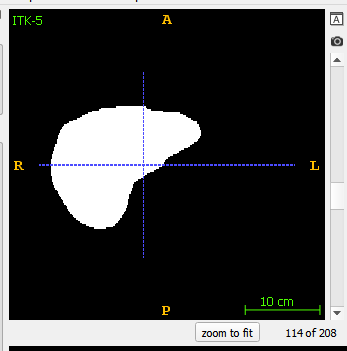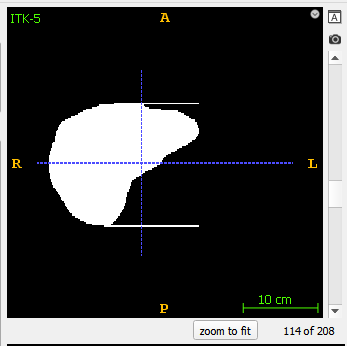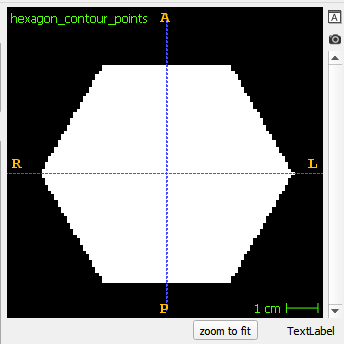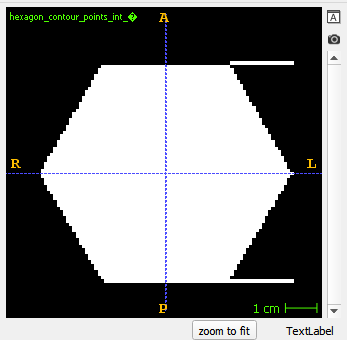# Problem in drawing geometrical object with ITK v5.0.0, but ITK v4 is good

Hi, I am working on a project which uses ITK to process medical images. Recently I upgraded ITK v4.13 to ITK v5.0.0 for the project, then I found something not working properly as before. Our function loads a list of points to draw a geometrical object on 2D image. The list of points is the contour of the object to draw. The function worked perfectly before upgraded to ITK v5, but the geometrical object is not drawn properly after upgraded to ITK v5:

Image before upgraded to ITK v5.0.0Image after upgraded to ITK v5.0.0I turned to try an ITK v5.0.0 example code Examples/Filtering/SpatialObjectToImage3.cxx in https://itk.org/Doxygen/html/Examples_2Filtering_2SpatialObjectToImage3_8cxx-example.html. The sample uses same classes and functions as that in my project.

The sample code generates a perfect hexagonIn the sample code, the code to generate the contour points of the hexagon is

``````...

// Software Guide : BeginCodeSnippet

using PolygonType = [itk::PolygonSpatialObject< Dimension >](https://itk.org/Doxygen/html/classitk_1_1PolygonSpatialObject.html);

...
// Software Guide : BeginLatex
//
// We populate the points of the polygon by computing the edges of a
// hexagon centered in the image.
//
// Software Guide : EndLatex
// Software Guide : BeginCodeSnippet

constexpr unsigned int numberOfPoints = 6;

typename [PolygonType::PointType](https://itk.org/Doxygen/html/namespaceitk_1_1GTest_1_1TypedefsAndConstructors_1_1Dimension2.html#a4e283d9983c57c5015a80d0b1d1cf09f) point;
typename [PolygonType::PointType::VectorType](https://itk.org/Doxygen/html/namespaceitk_1_1GTest_1_1TypedefsAndConstructors_1_1Dimension2.html#afc9b2e09ca815e90af469040c64abd94) radial;

radial = 0.0;
radial = 0.0;
radial = 0.0;

typename [PolygonType::PointType](https://itk.org/Doxygen/html/namespaceitk_1_1GTest_1_1TypedefsAndConstructors_1_1Dimension2.html#a4e283d9983c57c5015a80d0b1d1cf09f) center;

center = 50.0;
center = 50.0;
center = 0.0;
constexpr double radius = 40.0;

typename PolygonType::PolygonPointType polygonPoint;
for( unsigned int i=0; i < numberOfPoints; i++ )
{
const double angle = 2.0 * [itk::Math::pi](https://itk.org/Doxygen/html/namespaceitk_1_1Math.html#aec856bda469effc7a873b9baaae75583) * i / numberOfPoints;
radial = radius * std::cos( angle );
radial = radius * std::sin( angle );
point = center + radial;
polygonPoint.SetPositionInObjectSpace( point );
polygon->GetPoints().push_back( polygonPoint );
}

polygon->SetIsClosed(true);
polygon->Update();
...
``````

The generated hexagon contour points are: (90, 50), (70, 84.641), (30, 84.641), (10, 50), (30, 15.359), (70, 15.359).

However, when I modified the code to force the hexagon contour points to be: (90, 50), (70, 84), (30, 84), (10, 50), (30, 15), (70, 15), just remove the digits after the decimal point but still keep the coordinate values in double type, the image becomesDoes anyone have idea to avoid the comet like image to correctly draw the object from the contour points? Or is this a bug in ITK 5.0.0?

In my project, the coordinate value of all contour points have no digits after the decimal point. I temporarily add a value 0.0000000001 to x-y coordinate of all contour points to avoid the comet tail.

This looks like another instance of https://github.com/InsightSoftwareConsortium/ITK/issues/1082. @Stephen_Aylward can you take a look?

Yes, that is a similar case, the test point could be on the extra line

Will do!